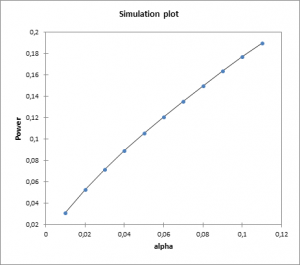# Statistical Power to compare variances

Ensure optimal power or sample size using power analysis. Power for the comparison of variances available in Excel using the XLSTAT statistical software.## Statistical Power analysis for the comparison of variances in XLSTAT

XLSTAT includes several tests to compare variances such as the test of comparison of the variances of two samples. XLSTAT can calculate the power or the number of observations required for a test based on Fisher's F distribution to compare variances. When testing a hypothesis using a statistical test, there are several decisions to take:

• The null hypothesis H0 and the alternative hypothesis Ha.
• The statistical test to use.
• The type I error also known as alpha. It occurs when one rejects the null hypothesis when it is true. It is set a priori for each test and is 5%.

The type II error or beta is less studied but is of great importance. In fact, it represents the probability that one does not reject the null hypothesis when it is false. We cannot fix it upfront, but based on other parameters of the model we can try to minimize it. The power of a test is calculated as 1-beta and represents the probability that we reject the null hypothesis when it is false. We therefore wish to maximize the power of the test. XLSTAT is able to compute the power (and beta) when other parameters are known. For a given power, it also allows to calculate the sample size that is necessary to reach that power.

The statistical power calculations are usually done before the experiment is conducted. The main application of power calculations is to estimate the number of observations necessary to properly conduct an experiment. XLSTAT allows you to compare two variances.

## Calculation for the Statistical Power analysis for the comparison of variances

The power of a test is usually obtained by using the associated non-central distribution. In that case, we use the F distribution. Several hypotheses can be tested, but the most common are the following (two-tailed):

• H0: The difference between the variances is equal to 0.
• Ha: The difference between the variances is different from 0.

The power computation will give the proportion of experiments that reject the null hypothesis. The calculation is done using the F distribution with the ratio of the variances as parameter and the sample sizes – 1 as degrees of freedom.

## Calculating sample size using the statistical power of a test

To calculate the number of observations required, XLSTAT uses an algorithm that searches for the root of a function. It is called the Van Wijngaarden-Dekker-Brent algorithm (Brent, 1973). This algorithm is adapted to the case where the derivatives of the function are not known. It tries to find the root of:

power (N) - expected_power

We then obtain the size N such that the test has a power as close as possible to the desired power.

## Effect size in power calculation

This concept is very important in power calculations. Indeed, Cohen (1988) developed this concept. The effect size is a quantity that will allow calculating the power of a test without entering any parameters but will tell if the effect to be tested is weak or strong. In the context of comparisons of means, the conventions of magnitude of the effect size are:

• d=0.2, the effect is small.
• d=0.5, the effect is moderate.
• d=0.8, the effect is strong.

XLSTAT allows entering the effect size directly.### analyze your data with xlstat

14-day free trial

Included in

Related features